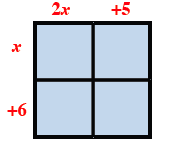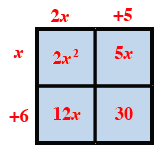Home > CC4 > Chapter 3 > Lesson 3.2.3 > Problem3-101

3-101.

Write an equation for each situation below showing that the area as a product is equal to the area as a sum. Homework Help ✎

1.  $12x$ $+1$ $x$ $-5$

• Review the Math Notes box in Lesson 3.2.2.

$(12x+1)(x−5)=12x^2−59x−5$

1.  $2m^2$ $-4m$ $-1$ $3m$ $+5$

• See part (a).

1. $(2x+5)(x+6)$

• Draw a generic rectangle and label the length and width.

$(2x + 5)(x + 6) = 2x^2 + 17x + 30$Fill in the grid by multiplying the length and width of each box.

Write an equation showing the product is the sum of the parts.
Area as a sum $= 2x^2 + 12x + 5x + 30 = 2x^2 + 17x + 30$
Area as a product = $(2x + 5)(x + 6)$

1. $( 3 - 5 y ) ( 2 + y )$

• See the help for part (c).